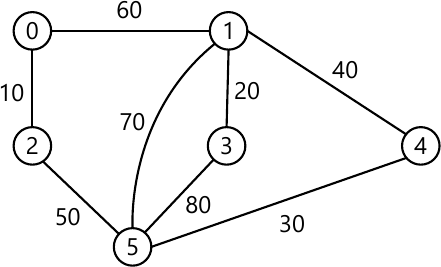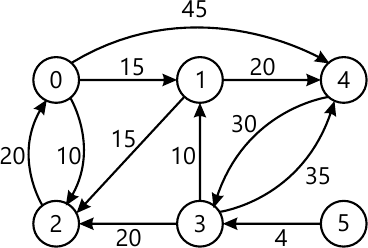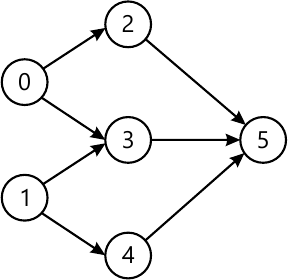MORE IN Design and Analysis of Algorithms
VTU Computer Science (Semester 4)
Design and Analysis of Algorithms
June 2013
Total marks: --
Total time: --
INSTRUCTIONS
(1) Assume appropriate data and state your reasons
(2) Marks are given to the right of every question
(3) Draw neat diagrams wherever necessary

1 (a) What is an algorithm? What are the properties of an algorithm? Explain with an example.
8 M
1 (b) Explain brute force method for algorithm design and analysis. Explain the brute force string matching algorithm with its efficiency.
8 M
1 (c) Express using asymptotic notation i) n! ii) 6×2n+n2
4 M

2 (a) Explain divide and conquer technique. Write the algorithm for binary search and find average case efficiency.
10 M
2 (b) What is stable algorithm? Is quick sort stable? Explain with example.
6 M
2 (c) Give an algorithm for merge sort.
4 M

3 (a) Explain the concept of greedy technique for Prim's algorithm. Obtain minimum cost spanning tree for the graph below Prim's algorithm.9 M
3 (b) Solve the following single source shortest path problem assuming vertex 5 as the source.9 M
3 (c) Define the following: i) Optimal solution; ii) Feasible solution
2 M

4 (a) Using Floyd's algorithm solve the all pair shortest problem for the graph whose weight matrix is given below: $\begin{bmatrix} 0 &\infty &3 &\infty \\2 &0 &\infty &\infty \\\infty &7 &0 &1 \\6 &\infty &\infty &0 \end{bmatrix}$
7 M
4 (b) Using dynamic programming, solve the following knapsack instance.
N=4. M=5
(W1, W2, W3, W4)= (2, 1, 3, 2)
( P1, P2, P3, P4)=(12, 10, 20, 15).
5 M
4 (c) Outline an exhaustive search algorithm to solve traveling salesman problem.
8 M

5 (a) Write and explain DFS and BFS algorithm with example.
8 M
5 (b) Obtain topologies sorting for the given diagram using source removal method.5 M
5 (c) Explain Horspool's string matching algorithm for a text that comprises letter and space (denoted by hyphen) i.e "JIM-SAW-ME-IN-BARBER-SHOP" with pattern "BARBER". Explain its working along with a neat table and algorithm to shift table.
7 M

6 (a) Define the following:
i) Class P
ii) Class NP
iii) NP complete problem
iv) NP hard problem
8 M
6 (b) Write the decision tree to sort the elements using selection sort and find the lower bound.
8 M
6 (c) What is numeric analysis?
2 M
6 (d) Brief overflow and underflow in numeric analysis algorithms.
2 M

7 (a) What is back tracking? Apply back tracking problem to solve the instance of the sum of subset problem: S={3,5,6,7} and d=15.
7 M
7 (b) With the help of a state space tree, solve the following instance of the knapsack problem by the branch and bound algorithm.
 Item Weight Value 1 2 3 4 4 7 5 3 40 42 25 12 Knapsack Capacity W=10
6 M
7 (c) Explain how backtracking is used for solving 4-Queen's problem. Show the state space table.
7 M

8 (a) What is prefix computation problem? Give the algorithm for prefix computation which uses
i) n processors; ii) n/log n, processors.
Obtain the time complexities of these algorithms.
10 M
8 (b) What is super linear speed up? Obtain the maximum speed up where P=10 and various values of f=0.5, 0.1, 0.001.
5 M
8 (c) What are the different ways resolving read and write conflicts?
5 M

More question papers from Design and Analysis of Algorithms